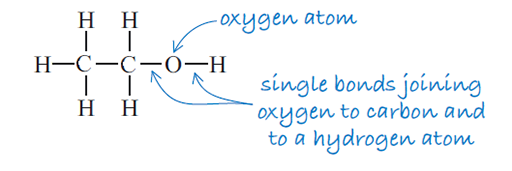The science of alcohol

Start this free course now. Just create an account and sign in. Enrol and complete the course for a free statement of participation or digital badge if available.

Free course

# 2 What is ethanol?

The proper chemical name for the alcohol in alcoholic drinks is ethanol. The chemical formula of ethanol is usually written as C2H5OH. There’s an important point to note here about how this formula is written.

• Can you see a possible problem if the formula were to be written as 2C5HOH?

• Use of the number 2 at the start of the formula implies that there are 2 atoms of every element which follows in this formula, which is not the case. This confusion is further increased by the use of the number 5 in front of the first H. This could imply that there are 5 O atoms as well in this formula.

The correct nomenclature for writing a chemical formula is to use numerical subscripts immediately following the atom, to show the correct number of atoms of that element in the formula.

So the formula C2H5OH makes it very clear that there are 2 carbon atoms, 5 hydrogen atoms, 1 oxygen atom (if there is no numerical subscript you can assume the value is 1) and 1 further H atom. In fact you can simplify this formula further by collecting numbers of like atoms (in this case the hydrogens) as you will see shortly.

The structural formula of ethanol is illustrated in Figure 3 and shows the order in which the atoms are joined together.Figure 3 The structural formula of ethanol
• How many atoms of each type are there in ethanol?

• There are two carbon atoms, six hydrogen atoms and one oxygen atom.

• How many atoms in total does this molecule contain?

• There are nine atoms in total.

• Does the formula C2H5OH tell you the order in which the atoms are bonded?

• The formula C2H5OH does not tell you the order in which the atoms are bonded. For that you need the structural formula (Figure 3) which shows how the atoms are arranged in the compound.

The structural formula is important because the way in which atoms bond together determines the size and shape of a molecule and how it interacts with the body. The size and shape of a molecule will determine, for example, whether or not it can dissolve in water and whether it is small enough to move across a cell membrane. This in turn affects the way the molecule interacts with the body.

SOA_1